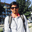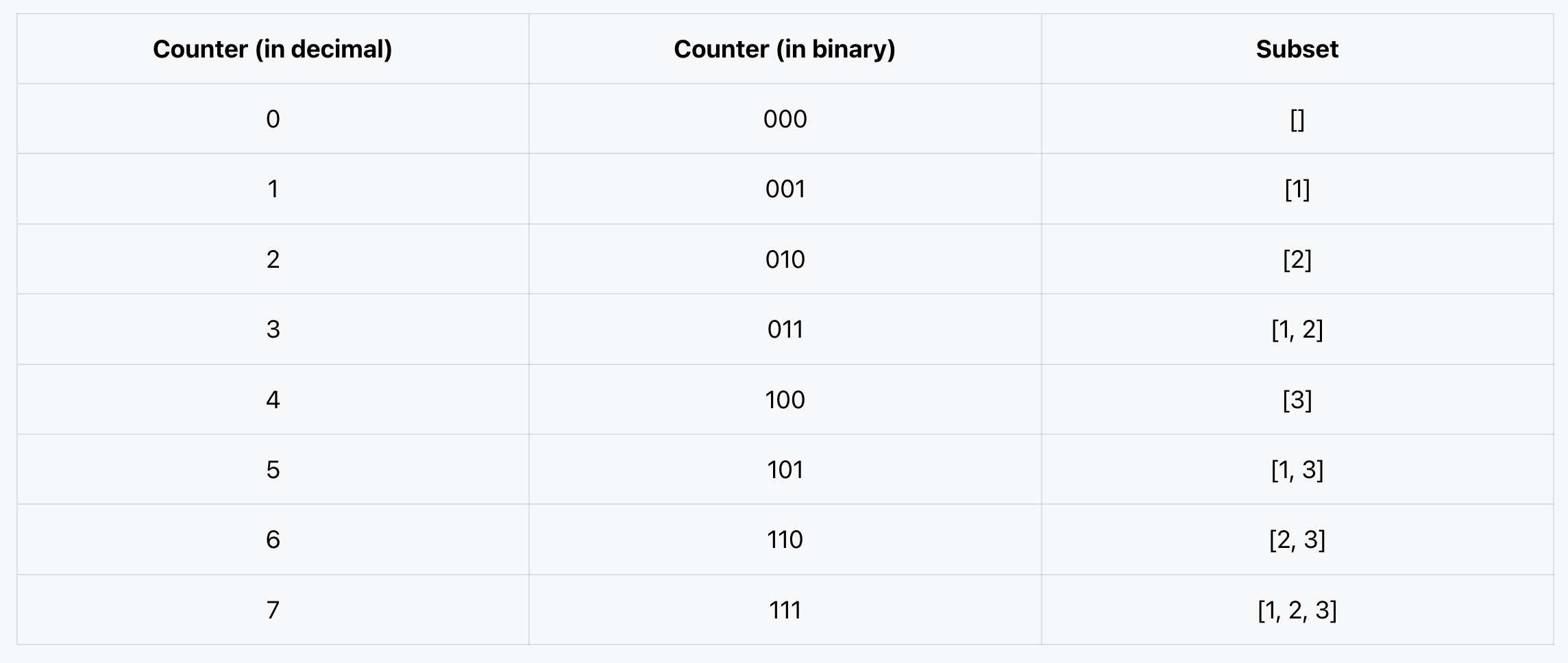Related Tags

bitwise
algorithms
communitycreator

# How to find nonidentical subsets of the given inputGopi Gorantala### Overview

In this shot, we discuss the subsets of a given input. This is one of the most popular questions asked in coding interviews.

Apple, Microsoft, Amazon, and Facebook are some of the companies that have asked this in their coding interviews.

### Problem statement

We need to write a program that finds all possible subsets (the power set) of a given input. The solution set must not contain duplicate subsets.

### Example 1

Input: [1, 2, 3]

Output: [[],,,[1,2],,[1,3],[2,3],[1,2,3]]


### Example 2

Input: 

Output: [[], ]


### Explanation

The subsets of any given input are equal to its power set. If input n = 3, then powerset => 2^n ​​= 2^3 = 8. We assume that the input has a length greater than or equal to 1.

Hint: We can use the left-shift operator to achieve this.

### Thought process

This program finds the power set of a given input using bitwise operations.

In general, if we have n elements, then the subsets are 2^​n. Therefore, for every possible case of having at least two elements, we can see that an element is present and not present in the subsets.

Let’s think of a solution that is iterative, uses bitwise operators, and generates the powerset.

Here is how we generate each subset using the outer-loop variable counter. The following table indicates how the value is generated based on the counter input.### Algorithm

We need to consider a counter variable that starts from 0 to 2^​n​​ - 1. We consider the binary representation for every value, and use the set bits in the binary representation to generate the corresponding subsets.

• If all set bits are 0, the corresponding subset is empty [].

• If the last bit is 1, we put 1 in the subset as .

### Steps

We use two loops here. The outer-loop starts from 0 to 2^​n​​ - 1, and the inner loop continues to input array length n.

In the inner loop, we conditionally check (counter & (1 << j)) != 0). If yes, then we print the corresponding element from an array.

### Solution

#include <iostream>
#include <vector>

using namespace std;

void subsets(vector<int>& nums){
int n = nums.size();
int powSize = 1 << n;;

for(int counter = 0; counter < powSize; counter++){
for(int j = 0; j < n; j++){
if((counter & (1 << j)) != 0){
cout<<"[" <<nums[j] << "]";
}
}
cout<<endl;
}
}

int main() {
vector<int> array = { 1, 2, 3 };
subsets(array);
}

Subsets/Power-set - C++ solution

### Complexity analysis

Time Complexity:

• O(n*2^n): The time complexity is n times the powerset.

Space Complexity:

• O(2^n): We storing 2^​n​​ subset elements in an array. So, the extra space is directly proportional to O(2^n​​).

I have also written a course on how to solve problems using bit manipulation. You can visit it here: Master Bit Manipulation for Coding Interviews.

RELATED TAGS

bitwise
algorithms
communitycreator

CONTRIBUTORGopi Gorantala
RELATED COURSES

View all Courses

Keep Exploring

Learn in-demand tech skills in half the time